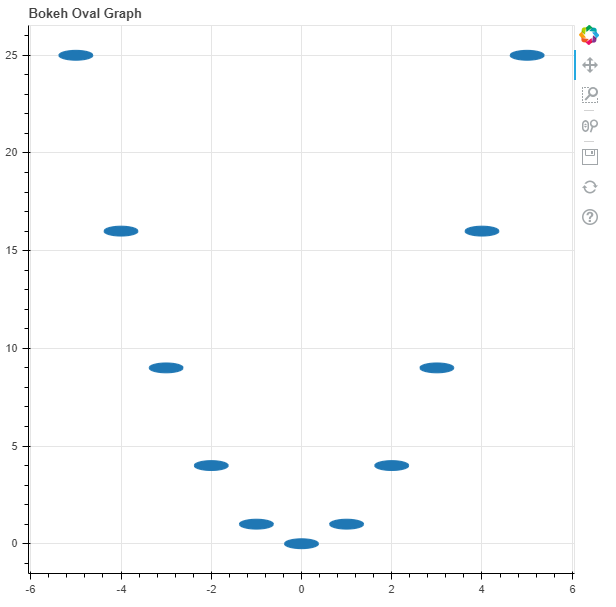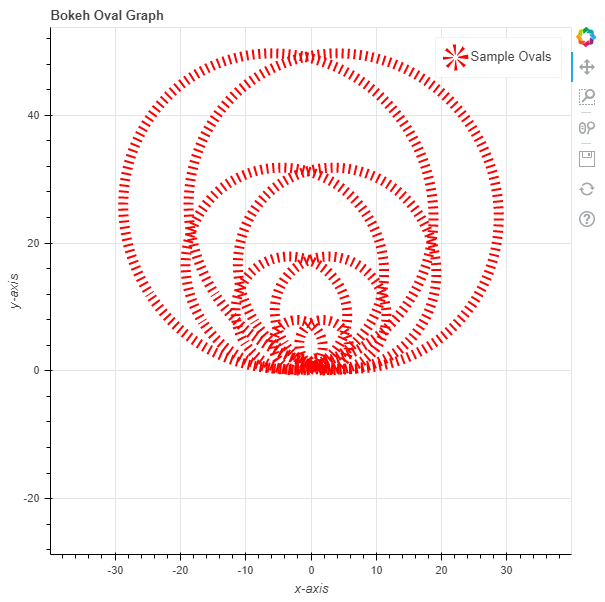# Python Bokeh – Plotting Ovals on a Graph

• Last Updated : 10 Jul, 2020

Bokeh is a Python interactive data visualization. It renders its plots using HTML and JavaScript. It targets modern web browsers for presentation providing elegant, concise construction of novel graphics with high-performance interactivity.

Bokeh can be used to plot ovals on a graph. Plotting ovals on a graph can be done using the `oval()` method of the `plotting` module.

## plotting.figure.oval()

Syntax : oval(parameters)

Parameters :

• x : x-coordinates of the center of the oval markers
• y : y-coordinates of the center of the oval markers
• width : width of the oval markers
• height : height of the oval markers
• width_units : unit of the width of the oval markers
• height_units : unit of the height of the oval markers
• angle : angle of rotation of the oval markers, default is 0
• angle_units : unit of the angle, default is rad
• fill_alpha : fill alpha value of the oval markers
• fill_color : fill color value of the oval markers
• line_alpha : percentage value of line alpha, default is 1
• line_cap : value of line cap for the line, default is butt
• line_color : color of the line, default is black
• line_dash : value of line dash such as : solid, dashed, dotted, dotdash, dashdot [default is solid ]
• line_dash_offset : value of line dash offset, default is 0
• line_join : value of line join, default in bevel
• line_width : value of the width of the line, default is 1
• name : user-supplied name for the model
• tags : user-supplied values for the model

Other Parameters :

• alpha : sets all alpha keyword arguments at once
• color : sets all color keyword arguments at once
• legend_field : name of a column in the data source that should be used
• legend_group : name of a column in the data source that should be used
• legend_label : labels the legend entry
• muted : determines whether the glyph should be rendered as muted or not, default is False
• name : optional user-supplied name to attach to the renderer
• source : user-supplied data source
• view : view for filtering the data source
• visible : determines whether the glyph should be rendered or not, default is True
• x_range_name : name of an extra range to use for mapping x-coordinates
• y_range_name : name of an extra range to use for mapping y-coordinates
• level : specifies the render level order for this glyph

Returns : an object of class `GlyphRenderer`

Example 1 : In this example we will be using the default values for plotting the graph.

 `# importing the modules``from` `bokeh.plotting ``import` `figure, output_file, show``     ` `# file to save the model``output_file(``"gfg.html"``)``     ` `# instantiating the figure object``graph ``=` `figure(title ``=` `"Bokeh Oval Graph"``)``   ` `# the points to be plotted``x ``=` `[``-``5``, ``-``4``, ``-``3``, ``-``2``, ``-``1``, ``0``, ``1``, ``2``, ``3``, ``4``, ``5``]``y ``=` `[i ``*``*` `2` `for` `i ``in` `x]``  ` `# plotting the graph``graph.oval(x, y,``           ``height ``=` `0.5``,``           ``width ``=` `1``)``   ` `# displaying the model``show(graph)`

Output :Example 2 : In this example we will be plotting the ovals with dotted lines alongside other parameters and the size of the hexagons are in proportion to their values.

 `# importing the modules``from` `bokeh.plotting ``import` `figure, output_file, show``    ` `# file to save the model``output_file(``"gfg.html"``)``    ` `# instantiating the figure object``graph ``=` `figure(title ``=` `"Bokeh Oval Graph"``)``  ` `# name of the x-axis``graph.xaxis.axis_label ``=` `"x-axis"``   ` `# name of the y-axis``graph.yaxis.axis_label ``=` `"y-axis"``  ` `# the points to be plotted``x ``=` `[``-``5``, ``-``4``, ``-``3``, ``-``2``, ``-``1``, ``0``, ``1``, ``2``, ``3``, ``4``, ``5``]``y ``=` `[i ``*``*` `2` `for` `i ``in` `x]``  ` `# width of the ovals``height ``=` `[i ``*` `2` `for` `i ``in` `y]`` ` `# angle of the ovals``width ``=` `[i ``*` `2.5` `for` `i ``in` `y]``  ` `# angle of the ovals``angle ``=` `10`` ` `# fill color value``fill_color ``=` `None`` ` `# color of the line``line_color ``=` `"red"``   ` `# type of line``line_dash ``=` `"dotted"``   ` `# offset of line dash``line_dash_offset ``=` `1``  ` `# width of the dashes``line_width ``=` `10``  ` `# name of the legend``legend_label ``=` `"Sample Ovals"``   ` `# plotting the graph``graph.oval(x, y,``          ``height ``=` `height,``          ``width ``=` `width,``          ``angle ``=` `angle,``          ``fill_color ``=` `fill_color,``          ``line_color ``=` `line_color,``          ``line_dash ``=` `line_dash,``          ``line_dash_offset ``=` `line_dash_offset,``          ``line_width ``=` `line_width,``          ``legend_label ``=` `legend_label)``  ` `    ` `# displaying the model``show(graph)`

Output :My Personal Notes arrow_drop_up